﻿ 基于TODIM的属性权重未知的混合多属性群决策方法

# 基于TODIM的属性权重未知的混合多属性群决策方法Hybrid Multiple Attribute Group Decision Making Method with Unknown Attribute Weights Based on TODIM

Abstract: With respect to hybrid multiple attribute group decision making problems with unknown attribute weights, based on normalizing the different types of evaluation values, this paper firstly develops a method for transforming crisp number, interval number and linguistic term into probabilistic linguistic term set. Then, by aggregating the individual decision matrices, the deviation maximization method is used to determine the weights of attributes, and the traditional TODIM method is extended to probabilistic linguistic environment. By constructing relative dominance matrix, the ranking of all feasible alternatives is determined according to the overall dominance degrees. Finally, a numerical example is provided to illustrate the applicability of the proposed method.

1. 引言

2. 基础理论

${\mu }_{\stackrel{˜}{a}}\left(x\right)=\left\{\begin{array}{l}\frac{x-{a}_{1}}{{a}_{2}-{a}_{1}},\text{\hspace{0.17em}}\text{\hspace{0.17em}}\text{ }{a}_{1} (1)

(1) 当 $i\le j$ 时， ${s}_{i}\le {s}_{j}$。例如，基数为5的语言术语集S可以表示为：S = {s0 = 很差, s1 = 差, s2 = 一般, s3 = 好, s4 = 很好}；

(2) 存在逆算子： $neg\left({s}_{i}\right)={s}_{j}$，且满足 $i+j=\tau$

$L\left(p\right)=\left\{{L}^{\left(x\right)}\left({p}^{\left(x\right)}\right)|{L}^{\left(x\right)}\in S,{p}^{\left(x\right)}\ge 0,x=1,2,3,\cdots ,#L\left(p\right),\underset{x=1}{\overset{#L\left(p\right)}{\sum }}{p}^{\left(x\right)}\le 1\right\},$ (2)

$\stackrel{˜}{L}\left(p\right)=\left\{{L}^{\left(x\right)}\left({\stackrel{˜}{p}}^{\left(x\right)}\right)|{L}^{\left(x\right)}\in S,{\stackrel{˜}{p}}^{\left(x\right)}\ge 0,x=1,2,\cdots ,#L\left(p\right),{\stackrel{˜}{p}}^{\left(x\right)}=p\left(x\right)/\underset{x=1}{\overset{#L\left(p\right)}{\sum }}p\left(x\right)\right\}.$ (3)

(1) ${L}_{1}\left(p\right)\oplus {L}_{\text{2}}\left(p\right)=\underset{{L}_{1}^{\left(x\right)}\in {L}_{1}\left(p\right),{L}_{2}^{\left(x\right)}\in {L}_{2}\left(p\right)}{\cup }\left\{{p}_{1}^{\left(x\right)}{r}_{1}^{\left(x\right)}\oplus {p}_{2}^{\left(x\right)}{r}_{2}^{\left(x\right)}\right\},$ (4)

(2) ${L}_{1}\left(p\right)\otimes {L}_{\text{2}}\left(p\right)=\underset{{L}_{1}^{\left(x\right)}\in {L}_{1}\left(p\right),{L}_{2}^{\left(x\right)}\in {L}_{2}\left(p\right)}{\cup }\left\{{\left({r}_{1}^{\left(x\right)}\right)}^{{p}_{1}^{\left(x\right)}}\otimes {\left({r}_{\text{2}}^{\left(x\right)}\right)}^{{p}_{\text{2}}^{\left(x\right)}}\right\},$ (5)

(3) $\lambda L\left(p\right)=\underset{{L}^{\left(x\right)}\in L\left(p\right)}{\cup }\left\{\lambda {p}^{\left(x\right)}{r}^{\left(x\right)}\right\}\text{ }\text{ },$ (6)

(4) ${\left(L\left(p\right)\right)}^{\lambda }=\underset{{L}^{\left(x\right)}\in L\left(p\right)}{\cup }\left\{{\left({r}^{\left(x\right)}\right)}^{\lambda {p}^{\left(x\right)}}\right\}\text{ }\text{ },$ (7)

$e\left(L\left(p\right)\right)=\underset{x=1}{\overset{#L\left(p\right)}{\sum }}{r}^{\left(x\right)}{p}^{\left(x\right)},$ (8)

$\delta \left(L\left(p\right)\right)=\underset{x=1}{\overset{#L\left(p\right)}{\sum }}{\left({r}^{\left(x\right)}-e\left(L\left(p\right)\right)\right)}^{2}{p}^{\left(x\right)},$ (9)

(1) 若 $G\left({L}_{1}\left(p\right)\right)>G\left({L}_{2}\left(p\right)\right)$，则 ${L}_{1}\left(p\right)>{L}_{2}\left(p\right)$

(2) 若 $G\left({L}_{1}\left(p\right)\right)=G\left({L}_{2}\left(p\right)\right)$，则 ${L}_{1}\left(p\right)={L}_{2}\left(p\right)$

$d\left({L}_{1}\left(p\right),{L}_{2}\left(p\right)\right)=\frac{\underset{x=1}{\overset{#L\left(p\right)}{\sum }}|{p}_{1}^{\left(x\right)}×{r}_{1}^{\left(x\right)}-{p}_{2}^{\left(x\right)}×{r}_{2}^{\left(x\right)}|}{#L\left(p\right)}.$ (10)

$\begin{array}{l}\text{PLWA}\left({L}_{1}\left(p\right),{L}_{2}\left(p\right),\cdots ,{L}_{n}\left(p\right)\right)\\ =\underset{i=1}{\overset{n}{\oplus }}\text{ }\text{ }{\omega }_{i}{L}_{i}\left(p\right)={\omega }_{1}{L}_{1}\left(p\right)\oplus {\omega }_{2}{L}_{2}\left(p\right)\oplus \cdots \oplus {\omega }_{n}{L}_{n}\left(p\right)\\ =\underset{{L}_{1}^{\left(x\right)}\in {L}_{1}\left(p\right)}{\cup }\left\{{\omega }_{1}{P}_{1}^{\left(x\right)}{r}_{1}^{\left(x\right)}\right\}\oplus \underset{{L}_{2}^{\left(x\right)}\in {L}_{2}\left(p\right)}{\cup }\left\{{\omega }_{2}{P}_{2}^{\left(x\right)}{r}_{2}^{\left(x\right)}\right\}\oplus \cdots \oplus \underset{{L}_{n}^{\left(x\right)}\in {L}_{n}\left(p\right)}{\cup }\left\{{\omega }_{n}{P}_{n}^{\left(x\right)}{r}_{n}^{\left(x\right)}\right\}\text{ }\text{ },\end{array}$ (11)

3. 基于TODIM的属性权重未知的混合多属性群决策方法

TODIM方法的主要思想是基于前景理论给出的价值函数，构造某一方案相对于其他方案的优势度函数，并根据总体优势度对所有备选方案进行排序。由于决策环境的不确定性与评价属性的多样性，为了准确地表达决策者的偏好信息，允许决策者利用不同类型的数据表示其对备选方案的评价信息。同时，考虑到决策者的心理行为和理性预期，提出了基于TODIM的属性权重未知的混合多属性群决策方法。首先，对不同数据类型的评价值进行规范化处理，将其转化到[0, 1]。然后，为了避免不同类型数据转化过程中的信息丢失，提出了将规范化评价值包括精确数、区间数、语言术语转化为概率语言术语集的方法。最后，在利用离差最大化思想确定属性权重的基础上，将传统TODIM方法拓展至概率语言环境下，通过计算各方案的总体优势度，确定方案的最终排序。

3.1. 不同数据类型的规范化方法

(1) 当属性 ${C}_{j}\in C$ ( $j\in {n}_{1}$ )时，精确数 ${z}_{ij}^{t}$ 的规范化处理方法为：

${e}_{ij}^{t}=\left\{\begin{array}{l}\frac{{z}_{ij}^{t}-\underset{\text{1}\le i\le m}{\mathrm{min}}\left\{{z}_{ij}^{t}\right\}}{\underset{\text{1}\le i\le m}{\mathrm{max}}\left\{{z}_{ij}^{t}\right\}-\underset{\text{1}\le i\le m}{\mathrm{min}}\left\{{z}_{ij}^{t}\right\}}\text{\hspace{0.17em}}\text{\hspace{0.17em}},j\in {C}_{B}\hfill \\ \frac{\underset{\text{1}\le i\le m}{\mathrm{max}}\left\{{z}_{ij}^{t}\right\}-{z}_{ij}^{t}}{\underset{\text{1}\le i\le m}{\mathrm{max}}\left\{{z}_{ij}^{t}\right\}-\underset{\text{1}\le i\le m}{\mathrm{min}}\left\{{z}_{ij}^{t}\right\}},j\in {C}_{C}\hfill \end{array},t=1,2,\cdots ,s;i=1,2,\cdots ,m;j\in {n}_{1}$ (12)

(2) 当属性 ${C}_{j}\in C$ ( $j\in {n}_{2}$ )时，区间数 ${z}_{ij}^{t}=\left[{z}_{1ij}^{t},{z}_{2ij}^{t}\right]$ 的规范化处理方法为：

${e}_{ij}^{t}=\left[{a}_{ij}^{t},{b}_{ij}^{t}\right]=\left\{\begin{array}{l}\left[\frac{{z}_{1ij}^{t}-\underset{1\le i\le m}{\mathrm{min}}\left\{{z}_{1ij}^{t}\right\}}{\underset{1\le i\le m}{\mathrm{max}}\left\{{z}_{2ij}^{t}\right\}-\underset{1\le i\le m}{\mathrm{min}}\left\{{z}_{1ij}^{t}\right\}},\frac{{z}_{2ij}^{t}-\underset{1\le i\le m}{\mathrm{min}}\left\{{z}_{1ij}^{t}\right\}}{\underset{1\le i\le m}{\mathrm{max}}\left\{{z}_{2ij}^{t}\right\}-\underset{1\le i\le m}{\mathrm{min}}\left\{{z}_{1ij}^{t}\right\}}\right],j\in {C}_{B}\hfill \\ \left[\frac{\underset{1\le i\le m}{\mathrm{max}}\left\{{z}_{2ij}^{t}\right\}-{z}_{2ij}^{t}}{\underset{1\le i\le m}{\mathrm{max}}\left\{{z}_{2ij}^{t}\right\}-\underset{1\le i\le m}{\mathrm{min}}\left\{{z}_{1ij}^{t}\right\}},\frac{\underset{1\le i\le m}{\mathrm{max}}\left\{{z}_{2ij}^{t}\right\}-{z}_{1ij}^{t}}{\underset{1\le i\le m}{\mathrm{max}}\left\{{z}_{2ij}^{t}\right\}-\underset{1\le i\le m}{\mathrm{min}}\left\{{z}_{1ij}^{t}\right\}}\right],j\in {C}_{C}\hfill \end{array},t=1,2,\cdots ,s;i=1,2,\cdots ,m;j\in {n}_{2}$ (13)

(3) 当属性 ${C}_{j}\in C$ ( $j\in {n}_{3}$ )时，每个语言术语 ${z}_{ij}^{t}$ 的隶属函数均与一个三角模糊数 $\left({z}_{1ij}^{t},{z}_{2ij}^{t},{z}_{3ij}^{t}\right)$ 相对应。如果三角模糊数不位于[0, 1]，则需要对其进行规范化处理：

${e}_{ij}^{t}=\left({a}_{ij}^{t},{b}_{ij}^{t},{c}_{ij}^{t}\right)=\left\{\begin{array}{l}\left(\frac{{z}_{1ij}^{t}}{\underset{1\le i\le m}{\mathrm{max}}\left\{{z}_{3ij}^{t}\right\}},\frac{{z}_{2ij}^{t}}{\underset{1\le i\le m}{\mathrm{max}}\left\{{z}_{2ij}^{t}\right\}},\frac{{z}_{3ij}^{t}}{\underset{1\le i\le m}{\mathrm{max}}\left\{{z}_{1ij}^{t}\right\}}\Lambda 1\right),j\in {C}_{B}\hfill \\ \left(\frac{\underset{1\le i\le m}{\mathrm{min}}\left\{{z}_{1ij}^{t}\right\}}{{z}_{3ij}^{t}},\frac{\underset{1\le i\le m}{\mathrm{min}}\left\{{z}_{2ij}^{t}\right\}}{{z}_{2ij}^{t}},\frac{\underset{1\le i\le m}{\mathrm{min}}\left\{{z}_{3ij}^{t}\right\}}{{z}_{1ij}^{t}}\Lambda 1\right),j\in {C}_{C}\hfill \end{array},t=1,2,\cdots ,s;i=1,2,\cdots ,m,j\in {n}_{3}$ (14)

3.2. 不同数据类型转化为概率语言术语集Table 1. Membership functions of linguistic terms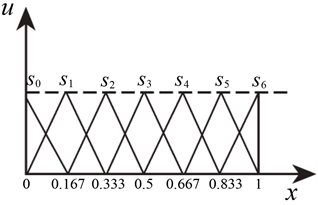Figure 1. Membership functions of linguistic terms

(1) 精确数转化概率语言术语集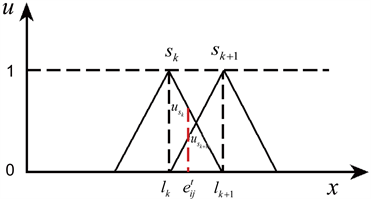Figure 2. Intersection of crisp numbers with linguistic terms sk and sk+1

${u}_{{s}_{k}}=\frac{{l}_{k+1}-{e}_{ij}^{t}}{{l}_{k+1}-{l}_{k}},\text{\hspace{0.17em}}\text{\hspace{0.17em}}{u}_{{s}_{k+\text{1}}}=\frac{{e}_{ij}^{t}-{l}_{k}}{{l}_{k+1}-{l}_{k}}.$ (15)

${p}_{{s}_{k}}=\frac{{u}_{{s}_{k}}}{{u}_{{s}_{k}}+{u}_{{s}_{k+\text{1}}}},\text{\hspace{0.17em}}\text{\hspace{0.17em}}{p}_{{s}_{k+1}}=\frac{{u}_{{s}_{k+\text{1}}}}{{u}_{{s}_{k}}+{u}_{{s}_{k+\text{1}}}}.$ (16)

(2) 区间数转化概率语言术语集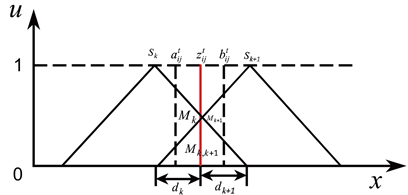Figure 3. Intersection of interval numbers with linguistic terms sk and sk+1

$A{F}_{k}\left({M}_{k,k+1}\right)=\frac{1}{2}\left[\left(1-\frac{{d}_{k}}{{d}_{k}+{d}_{k+1}}\right)+\frac{{M}_{k}}{{M}_{k}+{M}_{k+1}}\right],$ (17)

$A{F}_{k+\text{1}}\left({M}_{k,k+1}\right)=\frac{1}{2}\left[\left(1-\frac{{d}_{k+\text{1}}}{{d}_{k}+{d}_{k+1}}\right)+\frac{{M}_{k+\text{1}}}{{M}_{k}+{M}_{k+1}}\right],$ (18)

${P}_{{s}_{k}}=\frac{{M}_{k}+A{F}_{k}\left({M}_{k,k+1}\right)}{{M}_{k}+{M}_{k+1}+{M}_{k,k+1}},\text{\hspace{0.17em}}\text{\hspace{0.17em}}{P}_{{s}_{k+\text{1}}}=\frac{{M}_{k+\text{1}}+A{F}_{k+\text{1}}\left({M}_{k,k+1}\right)}{{M}_{k}+{M}_{k+1}+{M}_{k,k+1}}.$ (19)

(3) 语言术语转化概率语言术语集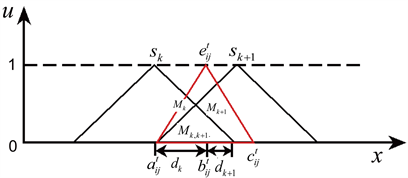Figure 4. Intersection of linguistic term with linguistic terms sk and sk+1

$A{F}_{k}\left({M}_{k,k+1}\right)=\frac{1}{2}\left[\left(1-\frac{{d}_{k}}{{d}_{k}+{d}_{k+1}}\right)+\frac{{M}_{k}}{{M}_{k}+{M}_{k+1}}\right],$ (20)

$A{F}_{k+\text{1}}\left({M}_{k,k+1}\right)=\frac{1}{2}\left[\left(1-\frac{{d}_{k+\text{1}}}{{d}_{k}+{d}_{k+1}}\right)+\frac{{M}_{k+\text{1}}}{{M}_{k}+{M}_{k+1}}\right],$ (21)

${P}_{{s}_{k}}=\frac{{M}_{k}+A{F}_{k}\left({M}_{k,k+1}\right)}{{M}_{k}+{M}_{k+1}+{M}_{k,k+1}},\text{\hspace{0.17em}}\text{\hspace{0.17em}}{P}_{{s}_{k+\text{1}}}=\frac{{M}_{k+\text{1}}+A{F}_{k+\text{1}}\left({M}_{k,k+1}\right)}{{M}_{k}+{M}_{k+1}+{M}_{k,k+1}}.$ (22)

a) 当语言术语 ${e}_{ij}^{t}$ 完全位于语言术语 ${s}_{k}$ 的隶属函数内时， ${e}_{ij}^{t}$ 属于 ${s}_{k}$ 的概率为1，对应的概率语言术语集为： ${e}_{ij}^{t}=\left({a}_{ij}^{t},{b}_{ij}^{t},{c}_{ij}^{t}\right)=\left\{\left({s}_{k},\text{1}\right)\right\}$

b) 当语言术语 ${e}_{ij}^{t}$ 仅与语言术语 ${s}_{k}$ 相交，且不与其他语言术语相交时，无论其是否完全位于语言术语 ${s}_{k}$ 的隶属函数内， ${e}_{ij}^{t}$ 属于 ${s}_{k}$ 的概率为1，对应的概率语言术语集为： ${e}_{ij}^{t}=\left({a}_{ij}^{t},{b}_{ij}^{t},{c}_{ij}^{t}\right)=\left\{\left({s}_{k},\text{1}\right)\right\}$

c) 当语言术语 ${e}_{ij}^{t}$ 同时与两个以上语言术语相交时，可以参照公式(20)和(21)分别计算 ${e}_{ij}^{t}$ 在各语言术语交叉面积的分配因子，以求得 ${e}_{ij}^{t}$ 属于每个语言术语的概率。

3.3. 确定属性的权重

Step 1：利用定义9中的PLWA算子对概率语言个体决策矩阵 ${L}^{t}\left(p\right)={\left({L}_{ij}^{t}\left(p\right)\right)}_{m×n}\text{\hspace{0.17em}}\left(t=\text{1},\text{2},\cdots ,s\right)$ 进行集结，求得概率语言群体决策矩阵 $L\left(p\right)={\left({L}_{ij}\left(p\right)\right)}_{m×n}$

Step 2：计算属性 ${C}_{j}\left(j=\text{1},\text{2},\cdots ,n\right)$ 下，方案Ai与其他方案 ${A}_{k}\left(k,i=\text{1},\text{2},\cdots ,m;k\ne i\right)$ 的评价值之间的偏差：

${\eta }_{ij}=\underset{k=1}{\overset{m}{\sum }}d\left({L}_{ij}\left(p\right),{L}_{kj}\left(p\right)\right)\text{ }\text{ },i=1,2,\cdots ,m;j=1,2,\cdots ,n.$ (23)

Step 3：计算属性 ${C}_{j}\left(j=\text{1},\text{2},\cdots ,n\right)$ 下，所有方案的偏差和：

${\eta }_{j}=\underset{i=1}{\overset{m}{\sum }}{\eta }_{ij},j=1,2,\cdots ,n.$ (24)

Step 4：利用离差最大化思想，确定属性 ${C}_{j}\left(j=\text{1},\text{2},\cdots ,n\right)$ 的权重向量 $\omega ={\left({\omega }_{1},{\omega }_{2},\cdots ,{\omega }_{n}\right)}^{\text{T}}$。根据离差最大化的思想，当某一属性下所有方案的评价值存在较大差异时，说明该属性包含的评价信息量较大，该属性应被赋予较大的权重，反之赋予较小的权重。当不存在差异，即所有方案的评价值相等时，该属性在决策过程中不起任何作用，因而权重为零。

${\omega }_{j}=\frac{{\eta }_{j}}{\underset{j=1}{\overset{n}{\sum }}{\eta }_{j}},j=1,2,\cdots ,n.$ (25)

3.4. 基于概率语言术语集的TODIM方法

Step 1：选取权重最大的属性作为参照权重，记作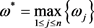，并计算属性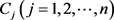的相对权重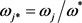Step 2：计算属性下，方案Ai相对于其他方案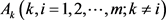的优势度：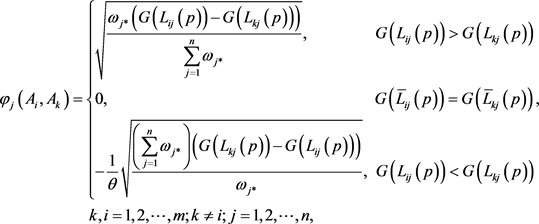(26)

Step 3：计算方案Ai相对于其他方案的综合优势度：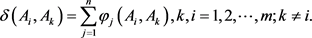(27)

Step 4：计算方案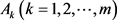的总体优势度：(28)

Step 5：按照方案总体优势度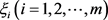由大到小的顺序，对备选方案进行排序。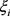越小，代表该方案越差；越大，代表该方案越好。

4. 算例分析Table 2. Membership functions of linguistic terms corresponding risk control levelTable 3. Individual decision matrices

Step 1：利用公式(12)~(14)对个体决策矩阵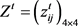进行规范化处理，求得规范化个体决策矩阵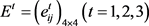，如表4所示。Table 4. Normalized individual decision matrices

Step 2：基于表1所示的概率语言术语集对应的语言术语的隶属函数，利用3.2节的不同数据类型转化为概率语言术语集的方法，将规范化个体决策矩阵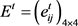转化为概率语言个体决策矩阵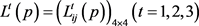，如表5所示。Table 5. Probabilistic linguistic individual decision matrices

Step 3：利用定义9中的PLWA算子对概率语言个体决策矩阵进行集结，求得概率语言群体决策矩阵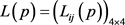，如表6所示。Table 6. Probabilistic linguistic group decision matrix

Step 4：根据离差最大化思想，利用公式(23)~(25)确定属性的权重，如下所示：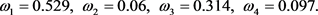Step 5：选取权重最大的属性C1作为参照权重，计算每个属性的相对权重，如下所示：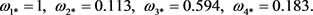Step 6：取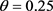，利用公式(26)和(27)计算方案Ai相对于其他方案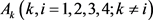的综合优势度，如下所示：Step 7：利用公式(28)，计算方案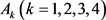的总体优势度，如下所示：Step 8：按照方案总体优势度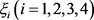由大到小的顺序，对备选方案进行排序：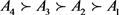。由此可得，A4为最满意方案。

5. 结论

 Zadeh, L.A. (1979) Fuzzy Set and Information Granularity. Advance in Fuzzy Set Theory & Application, 1, 3-18.

 Zadeh, L.A. (1972) A Fuzzy-Set-Theoretic Interpretation of Linguistic Hedges. Journal of Cybernetics, 2, 4-34.
https://doi.org/10.1080/01969727208542910

 Atanassov, K.T. and Rangasamy, P. (1986) Intuitionistic Fuzzy Sets. Fuzzy Sets & Systems, 20, 87-96.
https://doi.org/10.1016/S0165-0114(86)80034-3

 Torra, V. (2010) Hesitant Fuzzy Sets. International Journal of Intelligent Systems, 25, 529-539.
https://doi.org/10.1002/int.20418

 Lourenzutti, R. and Krohling, R.A. (2016) A Generalized TOPSIS Method for Group Decision Making with Heterogeneous Information in a Dynamic Environment. Information Sciences, 330, 1-18.
https://doi.org/10.1016/j.ins.2015.10.005

 赵萌, 张晨曦, 胡亦奇, 李刚. 基于愿景满意度函数的多属性群决策方法[J]. 中国管理科学, 2020, 28(2): 220-230.

 龚承柱, 李兰兰, 卫振锋, 诸克军. 基于前景理论和隶属度的混合型多属性决策方法[J]. 中国管理科学, 2014, 22(10): 122-128.

 张发明, 肖文星. 混合信息下的动态双激励评价机制设计及应用[J]. 中国管理科学, 2017, 25(12): 138-146.

 马金山. 指标及权重均为混合数据类型的广义灰靶决策方法[J]. 统计与决策, 2018, 34(7): 58-61.

 Peng, D.H., Gao, C.Y. and Wu, L.X. (2011) TOPSIS-Based Multi-Criteria Group Decision Making under Heterogeneous Information Setting. Advanced Materials Research, 378-379, 525-530.
https://doi.org/10.4028/www.scientific.net/AMR.378-379.525

 Gomes, L.F.A.M. and Lima, M.M.P.P. (1991) TODIM: Basic and Application to Multicriteria Ranking of Projects with Environmental Impacts. Foundations of Computing and Decision Sciences, 16, 113-127.

 Ren, Z.L., Xu, Z.S. and Wang, H. (2017) An Extended TODIM Method under Probabilistic Dual Hesitant Fuzzy Information and Its Application on Enterprise Strategic Assessment. IEEE International Conference on Industrial Engineering & Engineering Management, Singapore, 10-13 December 2017, 1464-1468.
https://doi.org/10.1109/IEEM.2017.8290136

 Sang, X. and Liu, X. (2016) An Interval Type-2 Fuzzy Sets-Based TODIM Method and Its Application to Green Supplier Selection. Journal of the Operational Research So-ciety, 67, 722-734.
https://doi.org/10.1057/jors.2015.86

 Lourenzutti, R., Krohling, R.A. and Reformat, M.Z. (2017) Choquet Based TOPSIS and TODIM for Dynamic and Heterogeneous Decision Making with Criteria Interaction. Information Sciences, 408, 41-69.
https://doi.org/10.1016/j.ins.2017.04.037

 Fan, Z.P., Zhang, X., Chen, F.D. and Liu, Y. (2013) Extended TODIM Method for Hybrid Multiple Attribute Decision Making Problems. Knowledge-Based Systems, 42, 40-48.
https://doi.org/10.1016/j.knosys.2012.12.014

 Tsaur, R.C. (2011) Decision Risk Analysis for an Interval TOPSIS Method. Applied Mathematics & Computation, 218, 4295-4304.
https://doi.org/10.1016/j.amc.2011.10.001

 Sevastjanov, P. and Tikhonenko, A. (2013) A Direct Interval Ex-tension of TOPSIS Method. Expert Systems with Applications, 40, 4841-4847.
https://doi.org/10.1016/j.eswa.2013.02.022

 Bohlender, R.B.G. (1986) Introduction to Fuzzy Arithmetic, Theory and Applications. Mathematics of Computation, 47, 762-763.
https://doi.org/10.2307/2008199

 Xu, Z. and Wang, H. (2017) On the Syntax and Semantics of Virtual Linguistic Terms for Information Fusion in Decision Making. Information Fusion, 34, 43-48.
https://doi.org/10.1016/j.inffus.2016.06.002

 Ertugrul, K.E. and Me-htap, D. (2015) An Integrated Fuzzy MCDM Approach for Supplier Evaluation and Selection. Computers & Industrial Engineering, 82, 82-93.
https://doi.org/10.1016/j.cie.2015.01.019

 Pang, Q., Xu, Z.S. and Wang, H. (2016) Probabilistic Linguistic Term Sets in Multi-Attribute Group Decision Making. Information Sciences, 369, 128-143.
https://doi.org/10.1016/j.ins.2016.06.021

 Zhang, X.F., Gou, X.J., Xu, Z.S. and Liao, H.C. (2019) A Projec-tion Method for Multiple Attribute Group Decision Making with Probabilistic Linguistic Term Sets. International Journal of Machine Learning and Cybernetics, 10, 2515-2528.
https://doi.org/10.1007/s13042-018-0886-6

Top